# Introduction to Network Analysis with NetworkX¶

by Georgy Lazarev (mlcourse slack: jorgy)

Systems are ubiquitous these days in various fields of science, industry and daily-life situations. Majority of them (to name few, social interactions, telephone and transport networks) can be represented as networks or graphs (sets of nodes and edges where nodes characterize certain objects and edges indicate some kind of connection between them) and therefore are suitable for analysis.

We might want to analyze relationships between participants or actor in those systems to get some valuable insights:

• Which nodes are more important (influencers in network)
• Pathfinding – determining shortest paths between certain nodes
• Structure - finding cliques, community detection (we'll discuss those terms later)

In this tutorial I'll introduce you to the NetworkX - Python package for 'the creation, manipulation, and study of the structure, dynamics, and functions of complex networks'. Important properties pointed out by developers:

• provides an interface to existing numerical algorithms and code written in C, C++, and FORTRAN, thus resulting in promising speed perfomance
• the ability to painlessly work with large nonstandard data sets.

Let's start with some basic things. At first you need to install NetworkX ('pip install networkx' or 'conda install ..' if you are working under Anaconda)

In :
#importing necessary packages
import networkx as nx
import matplotlib.pyplot as plt
import seaborn as sns
import pandas as pd
import csv
import numpy as np
%matplotlib inline
from collections import defaultdict
import warnings
warnings.filterwarnings('ignore')

In :
#creating empty Graph
G = nx.Graph()

#you can now add nodes and edges
#or creating list of nodes/edges in advance

In :
#setting attribute while creating node

#setting attribute to already existing node
G.node['John']['hobby']='writing music'
nx.set_node_attributes(G,'number',7)

#graph with random weghts
weights={}
for e in G.edges():
G[e][e]['weight']=np.random.random()

In :
print(G.edges())
print(G.edges(data=True))
#data=True prints attributes too

[('a', 1), ('a', 3), (2, 4)]
[('a', 1, {'weight': 0.6237962140335189}), ('a', 3, {'weight': 0.21626171858987386}), (2, 4, {'weight': 0.12734579259442702})]

In :
display(type(G))

networkx.classes.graph.Graph
In :
print(nx.info(G))

Name:
Type: Graph
Number of nodes: 6
Number of edges: 3
Average degree:   1.0000

In :
nx.draw(G,with_labels=True,node_size=900)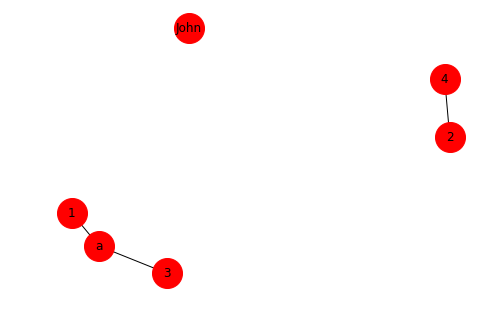It's probably worth mentioning that calling .draw without specifying pos argument will result in different layouts of nodes.

## Analysis of real graph¶

Here you can find real networks stored as graph representations (edgelist mostly) in .tsv format. For simplicity I picked quite small undirected graph, which represent frequent social interactions between 62 dolphins from community living off Doubtful Sound, a fjord in New Zealand. So this is sort of social network.

Reading graphs from files doesn't have unified way as data can be stored in different formats and graphs can be represented differently (adjacency matrix, edge lists, adjacency list). I'd recommend you go for reference here and here

In :
edges = []
with open('datasets/out.dolphins', 'r') as f:
edges.append(row[:2])
edges[:5]

Out:
[['9', '4'], ['10', '6'], ['10', '7'], ['11', '1'], ['11', '3']]
In :
#now create graph from edgelist we made in previous step
GD = nx.from_edgelist(edges)

In :
print(nx.info(GD))

Name:
Type: Graph
Number of nodes: 62
Number of edges: 159
Average degree:   5.1290

In :
nx.draw(GD,with_labels=True)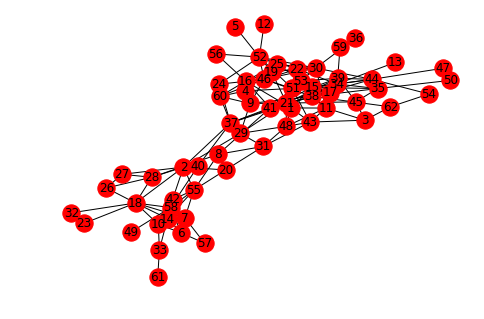There are other types of vizualization except usual one (especially useful when you are dealing with large graphs) - ArcPlot, MatrixPlot and etc. I'll show only one here - CircosPlot Let's install and import nice visualization package for NetworkX - nxviz.

In :
import nxviz as nv

In :
c=nv.CircosPlot(GD,node_labels=True)
c.draw()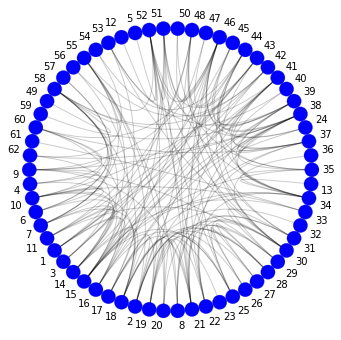That was fast :-) Now let's dive into analysis of our dolphins social graph

In :
#.has_edge method allows us to check presence of direct interaction between two nodes

GD.has_edge('40','37')

Out:
True
In :
print('shortest path from node 12 to 5 is of length', nx.shortest_path_length(GD,'12','5'),":", nx.shortest_path(GD,'12','5'))

shortest path from node 12 to 5 is of length 2 : ['12', '52', '5']


For every vertex in graph we can calculate its degree – number of adjacent (connected by an edge )vertices.

In :
display(list(GD.neighbors('9')))
print('node 9 has %d neighbors' %GD.degree()['9'])

['4', '21', '29', '38', '46', '60']
node 9 has 6 neighbors

In :
GD.degree()
#returns dictionary with all nodes

Out:
DegreeView({'9': 6, '4': 3, '10': 7, '6': 4, '7': 6, '11': 5, '1': 6, '3': 4, '14': 8, '15': 12, '16': 7, '17': 6, '18': 9, '2': 8, '19': 7, '20': 4, '8': 5, '21': 9, '22': 6, '23': 1, '25': 6, '26': 3, '27': 3, '28': 5, '29': 5, '30': 9, '31': 5, '32': 1, '33': 3, '34': 10, '13': 1, '35': 5, '36': 1, '37': 7, '24': 3, '38': 11, '39': 8, '40': 2, '41': 8, '42': 5, '43': 6, '44': 7, '45': 4, '46': 11, '47': 2, '48': 6, '50': 2, '51': 7, '52': 10, '5': 1, '12': 1, '53': 4, '54': 2, '55': 7, '56': 2, '57': 2, '58': 9, '49': 1, '59': 1, '60': 5, '61': 1, '62': 3})
In :
# find 5 nodes with largest amount of neighbors
sorted(dict(GD.degree()).items(), key=lambda x:x, reverse=True)[:5]

Out:
[('15', 12), ('38', 11), ('46', 11), ('34', 10), ('52', 10)]

That brings us to several types of centrality - metric for describing importance of a node

### Degree centrality¶

$$degree \ centrality = \frac {degree\ of\ node} {number\ of \ nodes\ in \ network}$$
In :
#We create dictionary with nodes and their degree centralities
degcent=nx.degree_centrality(GD) #for getting dictionary with nodes and
#their degree centralities as keys and values respectively

print("Networkx degree centrality for node 16:",degcent['16'])

Networkx degree centrality for node 16: 0.11475409836065574


Let's see 5 nodes with largest degree centrality:

In :
sorted(degcent.items(), key=lambda x: x, reverse=True)[:5]

Out:
[('15', 0.19672131147540983),
('38', 0.18032786885245902),
('46', 0.18032786885245902),
('34', 0.1639344262295082),
('52', 0.1639344262295082)]
In :
#we'll store node with the largest degree centraliy as 'nld '
nld=sorted(degcent.items(), key=lambda x: x, reverse=True)[:1]


Here goes sort of vizualization of this node:

In :
palette = sns.color_palette('coolwarm',2).as_hex()
palette

Out:
['#aac7fd', '#f7b89c']
In :
#this list wi'll be used in .draw call.
node_colors=[palette if n!=nld else palette for n in GD.nodes() ]

In :
nx.draw(GD,node_color=node_colors,with_labels=True)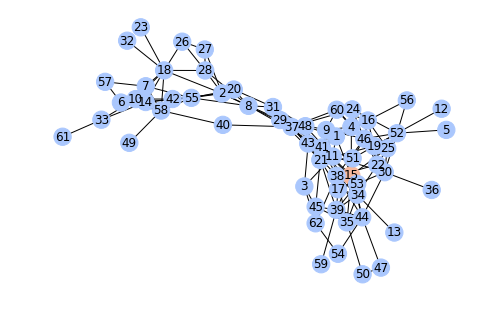As mentioned above you can set attributes to nodes. Let's say we want each node to keep its degree as (one of) attribute:

In :
for n in GD.nodes():
GD.node[n]['degree centrality'] = degcent[n]


Now we can use it in customizing our vizualization:

In :
c=nv.CircosPlot(GD,node_order='degree centrality',node_labels=True)
c.draw()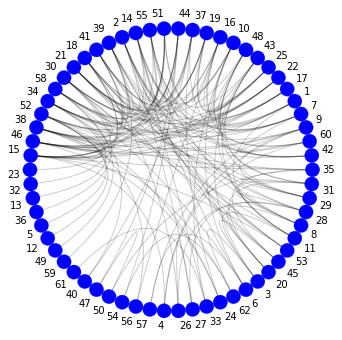### Closeness centrality¶

$$closeness \ centrality = \frac {N\ -\ 1} {sum\ of \ distances\ to \ each \ node \ from \ current \ one}$$

where $N =$ number of nodes

Compares how many steps (edges) it would take to reach every other node in a network (using shortest paths obviously). Intuitively you can think of this as of 'average distance' to all other nodes.

Degree centrality takes into account only direct connections thus deluding analyst sometimes as current node can be central only in local neighborhood. That's why closeness centrality is important.

In case pf being not fully connected, this algorithm computes the closeness centrality for each connected component separately. By the way, you can see connected parts of your graph this way (in our case graph is itself connected):

In :
for comp in nx.connected_components(GD):
print(comp)

{'9', '58', '35', '23', '19', '14', '33', '1', '31', '21', '60', '16', '41', '42', '10', '24', '56', '40', '2', '5', '3', '27', '51', '34', '61', '62', '59', '4', '32', '29', '18', '45', '43', '49', '47', '8', '26', '13', '46', '7', '57', '54', '6', '22', '52', '12', '44', '39', '15', '25', '53', '37', '28', '30', '55', '38', '11', '50', '17', '20', '48', '36'}

In :
#calculating closeness centrality for each node
clocent = nx.closeness_centrality(GD)

In :
sorted(clocent.items(), key=lambda x: x, reverse=True)[:5]

Out:
[('37', 0.4178082191780822),
('41', 0.40397350993377484),
('38', 0.39869281045751637),
('21', 0.391025641025641),
('15', 0.3765432098765432)]
In :
for n in GD.nodes():
GD.node[n]['closeness centrality'] = clocent[n]

In :
nlcc=sorted(clocent.items(), key=lambda x: x, reverse=True)[:1]
palette = sns.color_palette('coolwarm',2).as_hex()
node_colors=[palette if n!=nlcc else palette for n in GD.nodes() ]

In :
nx.draw(GD,node_color=node_colors,with_labels=True)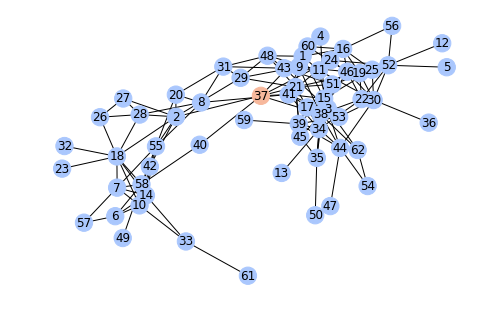### Betweeness Centrality¶

We can calculate shortest path for each pair of node (if they belong to one connected component obviously). So betweeness centrality of a node indicates to how often this current node becomes a part of all shortest paths.

$$betweeness \ centrality = \frac {number \ of \ shortest \ paths \ passing \ throught \ current \ node } {totul \ number \ of \ shortest \ paths}$$

Intuitively betweeness centrality can be thought of as measure of node influence in network. For example, members of a group with 'big' influence can be useful in terms of spreading a message/information. As an other example - in telecommunication network a node with largest betweeness centrality has biggest control due to amount information transferring through it.

In :
betcent = nx.betweenness_centrality(GD)
for n in GD.nodes():
GD.node[n]['betweeness centrality'] = betcent[n]

sorted(betcent.items(), key=lambda x: x, reverse=True)[:5]

Out:
[('37', 0.24823719602893804),
('2', 0.213324435532811),
('41', 0.14314951834261747),
('38', 0.13856978865859435),
('8', 0.11823861926938342)]
In :
nlbc=sorted(clocent.items(), key=lambda x: x, reverse=True)[:1]
palette = sns.color_palette('coolwarm',2).as_hex()
node_colors=[palette if n!=nlbc else palette for n in GD.nodes() ]

In :
nx.draw(GD,node_color=node_colors,with_labels=True)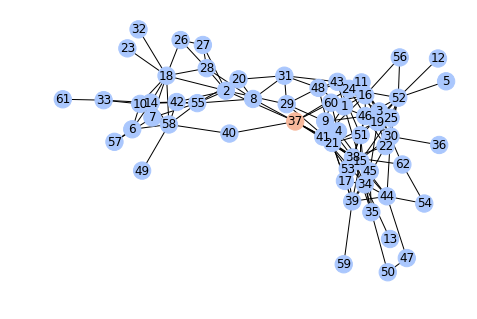$Betweeness \ centrality \ for \ edges$

I guess, this term doesn't need an explanation as its meaning is almost the same as for node

In :
betcent_e=nx.edge_betweenness_centrality(GD)
sorted(betcent_e.items(), key=lambda x: x, reverse=True)[:5]

Out:
[(('2', '37'), 0.14963002250231947),
(('8', '41'), 0.11583748083552335),
(('18', '2'), 0.09736232147230936),
(('37', '38'), 0.09553804999570528),
(('37', '40'), 0.09162347458612637)]
In :
for e in GD.edges():
GD[e][e]['betweeness centrality']=betcent_e[e]

In :
#the bigger is betweeness centrality - the darker are edges
c=nv.CircosPlot(GD,edge_color='betweeness centrality')
c.draw()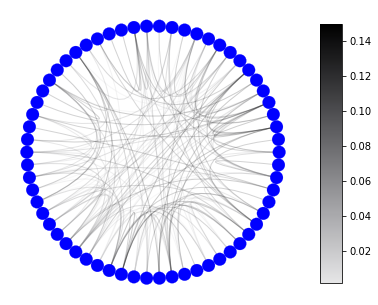In :
elbc=sorted(betcent_e.items(), key=lambda x: x, reverse=True)[:1]
palette=['#bd3c14','#009688']
edge_colors=[palette if e!=elbc else palette for e in GD.edges() ]

In :
nx.draw(GD,edge_color=edge_colors,with_labels=True,node_color='#999999')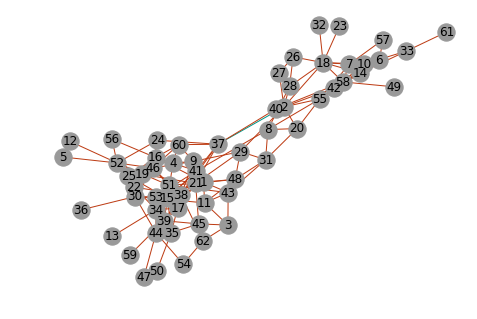The green edge is the one with the largest betweeness centrality. Colors are quite distinguishable, I hope :-)

### Eigenvector Centrality¶

OK, I tried to avoid this but here is real formula without words:

$$Ax = \lambda x$$

A is adjacency matrix of graph, (one of the (if not the one) most-used representation of graph) - NxN matrix where values in intersections of columns and rows corresponds to connection between respective nodes. Non-zero values stand for connection between nodes (in case of unweighted graph 1 is the only option) and zero does mean nodes are not directly connected. $\lambda$ is egenvalue of A and the largest eigenvalue associated with the eigenvector of the adjacency matrix A

Importance of a node depends on the importance of its neighbors.

In :
eigcent = nx.eigenvector_centrality_numpy(GD)
sorted(eigcent.items(), key=lambda x: x, reverse=True)[:5]

Out:
[('15', 0.3157828494923709),
('38', 0.300562054677549),
('46', 0.2850052152846933),
('34', 0.2810986921916837),
('51', 0.2176917909396778)]
In :
for n in GD.nodes():
GD.node[n]['eigenvector centrality'] = eigcent[n]

In :
#you can use this function for colouring nodes according to their values of given attribute
def attribute_color(G,attribute):
attrs=[G.node[n][attribute] for n in G.nodes()]
uattrs=sorted(list(set(attrs)))
palette = sns.color_palette('Blues',len(uattrs)).as_hex()
colmap=dict(zip(uattrs,palette))
node_colors=[colmap[at] for at in attrs]
nx.draw(G,node_color=node_colors,with_labels=True)


Let's see all four types of centrality in one picture. Darker shades of blue corresponds to bigger values of metric

In :
fig = plt.figure(figsize=(12, 12))

plt.subplot(221)
attribute_color(GD,'degree centrality')
plt.subplot(222)
attribute_color(GD,'closeness centrality')
plt.subplot(223)
attribute_color(GD,'betweeness centrality')
plt.subplot(224)
attribute_color(GD,'eigenvector centrality')